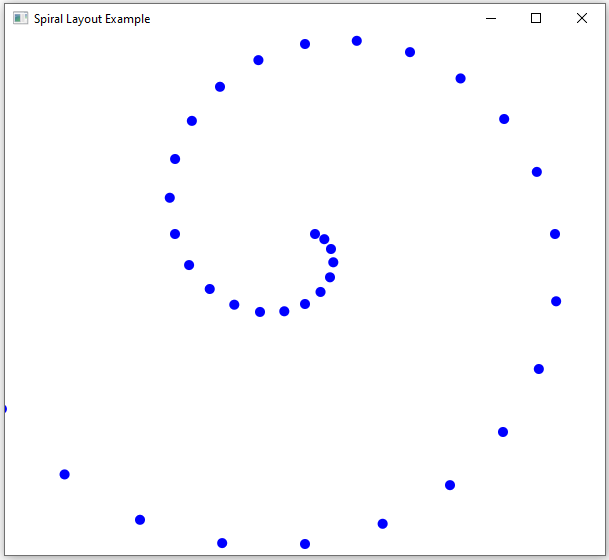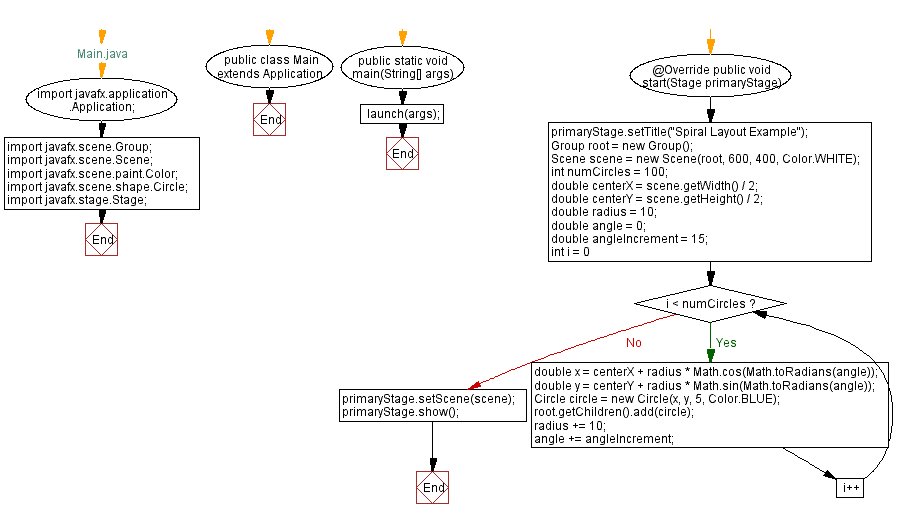﻿ JavaFX Spiral layout design

# JavaFX Spiral layout design

## JavaFx Layout Management: Exercise-10 with Solution

Write a JavaFX application that implements a custom layout for arranging items in a spiral fashion.

Sample Solution:

JavaFx Code:

``````//Main.java

import javafx.application.Application;
import javafx.scene.Group;
import javafx.scene.Scene;
import javafx.scene.paint.Color;
import javafx.scene.shape.Circle;
import javafx.stage.Stage;

public class Main extends Application {

public static void main(String[] args) {
launch(args);
}

@Override
public void start(Stage primaryStage) {
primaryStage.setTitle("Spiral Layout Example");
Group root = new Group();
Scene scene = new Scene(root, 600, 400, Color.WHITE);

int numCircles = 100;
double centerX = scene.getWidth() / 2;
double centerY = scene.getHeight() / 2;
double angle = 0;
double angleIncrement = 15;

for (int i = 0; i < numCircles; i++) {

Circle circle = new Circle(x, y, 5, Color.BLUE);

angle += angleIncrement;
}

primaryStage.setScene(scene);
primaryStage.show();
}
}
```
```

In the exercise above, we use the 'Math.cos' and 'Math.sin' functions to calculate the x and y positions of each circle in a spiral pattern. The 'angle' variable is incremented to change the angle of placement, and the 'radius' is adjusted to control the spacing between circles.

Note: Adjust the values and parameters to create different spiral patterns.

Sample Output:

``````

Flowchart:Java Code Editor:

What is the difficulty level of this exercise?

Test your Programming skills with w3resource's quiz.

﻿